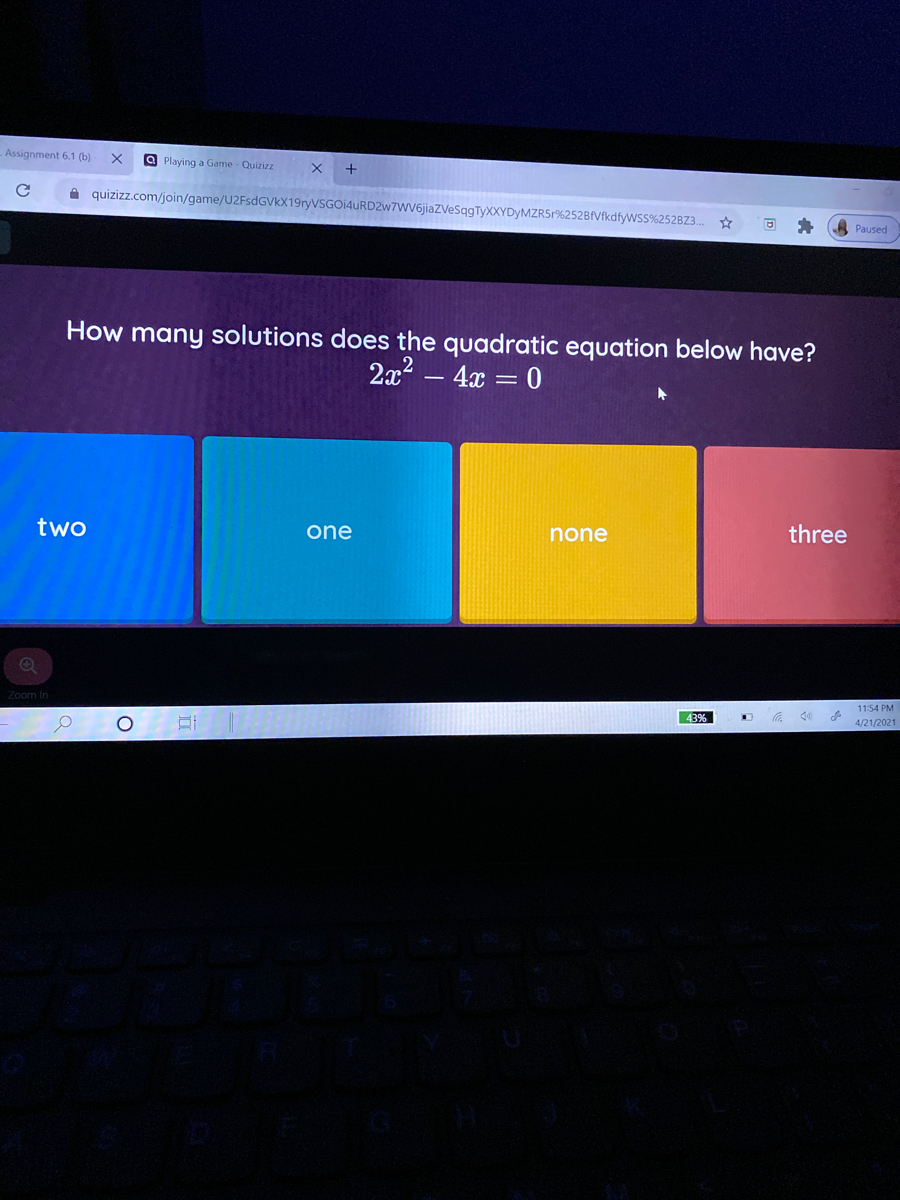# How many solutions does the quadratic equation below have? 2x2 – 4x = 0 two one none three

Question
how many solutions does the quadratic equation below have? 2x^2 - 4x = 0help_outlineImage TranscriptioncloseHow many solutions does the quadratic equation below have? 2x2 – 4x = 0 two one none three fullscreen

### Want to see this answer and more?

Experts are waiting 24/7 to provide step-by-step solutions in as fast as 30 minutes!*

*Response times may vary by subject and question complexity. Median response time is 34 minutes for paid subscribers and may be longer for promotional offers.
Tagged in
Math
Algebra

### Equations and In-equations